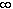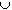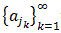#Interactive Real Analysis

Next | Previous | Glossary | Map

## 5. Topology

### 5.1. Open and Closed Sets

In the previous chapters we dealt with collections of points: sequences and series. Each time, the collection of points was either finite or countable and the most important property of a point, in a sense, was its location in some coordinate or number system. Now will deal with points, or more precisely with sets of points, in a more abstract setting. The location of points relative to each other will be more important than their absolute location, or size, in a coordinate system. Therefore, concepts such as addition and multiplication will not work anymore, and we will have to start, in a sense, at the beginning again.

All of the previous sections were, in effect, based on the natural numbers. Those numbers were postulated as existing and all other properties - including other number systems - were deduced from those numbers and a few principles of logic.

We will now proceed in a similar way: first, we need to define the basic objects we want to deal with, together with their most elementary properties. Then we will develop a theory of those objects and called it topology.

 Definition 5.1.1: Open and Closed Sets A set UR is called open, if for each xU there exists an> 0 such that the interval ( x -, x +) is contained in U. Such an interval is often called an- neighborhood of x, or simply a neighborhood of x. A set F is called closed if the complement of F, R \ F, is open.
 Examples 5.1.2: Which of the following sets are open, closed, both, or neither ?The intervals (-3, 3), [4, 7], (-4, 5], (0,) and [0,)The sets R (the whole real line) and 0 (the empty set)The set {1, 1/2, 1/3, 1/4, 1/5, ...} and {1, 1/2, 1/3, 1/4, ...}{0}
It is fairly clear that when combining two open sets (either via union or intersection) the resulting set is again open, and the same statement should be true for closed sets. What about combining infinitely many sets ?
 Proposition 5.1.3: Unions of Open Sets, Intersections of Closed Sets Every union of open sets is again open. Every intersection of closed sets is again closed. Every finite intersection of open sets is again open Every finite union of closed sets is again closed.

How complicated can an open or closed set really be ? The basic open (or closed) sets in the real line are the intervals, and they are certainly not complicated. As it will turn out, open sets in the real line are generally easy, while closed sets can be very complicated.

The worst-case scenario for the open sets, in fact, will be given in the next result, and we will concentrate on closed sets for much of the rest of this chapter.

 Proposition 5.1.4: Characterizing Open Sets Let UR be an arbitrary open set. Then there are countably many pairwise disjoint open intervals Un such that U =Un
Next we need to establish some relationship between topology and our previous studies, in particular sequences of real numbers. We shall need the following definitions:
 Definition 5.1.5: Boundary, Accumulation, Interior, and Isolated Points Let S be an arbitrary set in the real line R. A point bR is called boundary point of S if every non-empty neighborhood of b intersects S and the complement of S. The set of all boundary points of S is called the boundary of S, denoted by bd(S). A point sS is called interior point of S if there exists a neighborhood of S completely contained in S. The set of all interior points of S is called the interior, denoted by int(S). A point tS is called isolated point of S if there exists a neighborhood U of t such that US = {t}. A point rS is called accumulation point, if every neighborhood of r contains infinitely many distinct points of S.
 Examples 5.1.6:What is the boundary and the interior of (0, 4), [-1, 2], R, and O ? Which points are isolated and accumulation points, if any ?Find the boundary, interior, isolated and accumulation points, if any, for the set {1, 1/2, 1/3, ... }{0}
Here are some results that relate these various definitions with each other.
 Proposition 5.1.7: Boundary, Accumulation, Interior, and Isolated Points Let SR. Then each point of S is either an interior point or a boundary point. Let SR. Then bd(S) = bd(R \ S). A closed set contains all of its boundary points. An open set contains none of its boundary points. Every non-isolated boundary point of a set SR is an accumulation point of S. An accumulation point is never an isolated point.
Finally, here is a theorem that relates these topological concepts with our previous notion of sequences.

 Theorem 5.1.8: Closed Sets, Accumulation Points, and Sequences A set SR is closed if and only if every Cauchy sequence of elements in S has a limit that is contained in S. Every bounded, infinite subset of R has an accumulation point. If S is closed and bounded, andis any sequence in S, then there exists a subsequenceofthat converges to an element of S.
Next | Previous | Glossary | Map